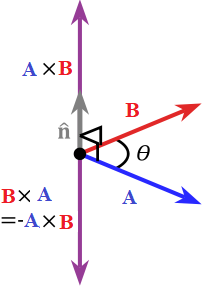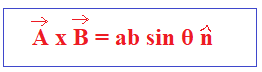# Cross Product

Cross product is the binary operation on two vectors in three dimensional space. It again results in a vector which is perpendicular to both the vectors. Cross product of two vectors is calculated by right hand rule.

Right hand rule is nothing but the resultant of any two vectors is perpendicular to the other two vectors. Using cross product, we can also find the magnitude of the resulting vector.

## Definition

Vector product or cross product of two vectors

$$\begin{array}{l}\vec{A}\end{array}$$
and
$$\begin{array}{l}\vec{B}\end{array}$$
is denoted by
$$\begin{array}{l}\vec A \times \vec B\end{array}$$
and its resultant vector is perpendicular to the vectors
$$\begin{array}{l}\vec{A}\end{array}$$
and
$$\begin{array}{l}\vec{B}\end{array}$$
.Key points to remember:

• Cross product of two vectors is always a vector quantity.
• In vector product, the resulting vector contains a negative sign if the order of vectors is changed.
• Direction of
$$\begin{array}{l}\vec A \times \vec B\end{array}$$
is always perpendicular to the plane containing
$$\begin{array}{l}\vec{A}\end{array}$$
and
$$\begin{array}{l}\vec{B}\end{array}$$
.
• Cross product of any two linear vectors is always a null vector.

## Cross Product Formula

If

$$\begin{array}{l}\theta\end{array}$$
is the angle between the given vectors, then the formula is given by

$$\begin{array}{l}A \times B = AB\ sin \theta\end{array}$$Where

$$\begin{array}{l}\hat n\end{array}$$
is the unit vector.

## Cross Product of Two Vectors

Cross product of two vectors is indicated like,

$$\begin{array}{l}\vec X \times \vec Y = |\vec X| . |\vec Y| \sin \theta\end{array}$$

Let us take any two vectors

$$\begin{array}{l}\vec X = x \vec i + y \vec j + z \vec k\end{array}$$
and
$$\begin{array}{l}\vec y = a \vec i + b \vec j + c \vec k\end{array}$$

So, cross product of these two vectors can be defined by matrices form, also called determinant form.

$$\begin{array}{l}\vec X \times \vec Y = \vec i (yc – zb) – \vec j (xc – za) + \vec k (xb – ya)\end{array}$$
.

## Proof

Let

$$\begin{array}{l}A = ai + bj + ck\end{array}$$
and
$$\begin{array}{l}B = di + ej + fk\end{array}$$
are the two given vectors, then

$$\begin{array}{l}\left \| A\times B \right \|= \sqrt{(bf-ec)^{2}+ (dc-fa)^{2}+ (ae-bd)^{2}}\end{array}$$

=

$$\begin{array}{l}\sqrt{b^{2}f^{2}+e^{2}c^{2}-2bcef+d^{2}c^{2}+ f^{2}a^{2}-2acdf + a^{2}e^{2}+ b^{2}d^{2}-2abde}\end{array}$$

=

$$\begin{array}{l}\sqrt{(a^{2}+b^{2}+c^{2})(d^{2}+e^{2}+f^{2})\left \{ 1-\frac{\left ( (ai+bj+ck).(di+ej+fk) \right )^{2}}{(a^{2}+b^{2}+c^{2})(d^{2}+e^{2}+f^{2})} \right \}}\end{array}$$

=

$$\begin{array}{l}\left \| A \right \|.\left \| B \right \|\sqrt{1- cos ^{2}\theta}\end{array}$$

=

$$\begin{array}{l}\left \| A \right \|.\left \| B \right \|sin \theta\end{array}$$

This shows that the magnitude of the cross product is the area of the parallelogram which is formed by the use of given two vectors.

Direction of cross product

The direction of the cross product of two non zero parallel vectors a and b is given by the right hand thumb rule. In your right hand, point your index finger along the vector a and point your middle finger along vector b, then the thumb gives the direction of the cross product.

## Triple Cross Product

The product of three vectors is called the triple product. In other words, the cross product of one vector with the cross product of another two vectors.

If we have three vectors,

$$\begin{array}{l}\vec A, \vec B\;\ and\;\ \vec {C}\end{array}$$
, then the vector triple product is denoted as follows:

A × (B × C) = (A . C) B − (A . B) C

(A × B) × C = −C × (A × B) = −(C . B) A + (C . A) B

## Cross Product Rules

Anti-Commutative Property

The Cross product is an anti commutative property

It means

$$\begin{array}{l}\vec A \times \vec B = – \vec B \times \vec A\end{array}$$

Let

$$\begin{array}{l}\vec A = a_1 \vec i + a_2 \vec j + a_3 \vec k\end{array}$$
and
$$\begin{array}{l}\vec B = b_1 \vec i + b_2 \vec j + b_3 \vec k\end{array}$$
,

$$\begin{array}{l}\vec A \times \vec B\end{array}$$
=
$$\begin{array}{l}\begin{vmatrix} i& j& k&\\ a_1& a_2& a_3& \\ b_1& b_2& b_3& \end{vmatrix}\end{array}$$

=

$$\begin{array}{l}(a_2b_3 – b_2a_3)\vec i – (a_1b_3 – b_1a_3 ) \vec j + (a_1b_2 – a_2b_1) \vec k\end{array}$$
,

$$\begin{array}{l}\vec B \times \vec A\end{array}$$
=
$$\begin{array}{l}\begin{vmatrix} i& j& k&\\ b_1& b_2& b_3& \\ a_1& a_2& a_3& \end{vmatrix}\end{array}$$

=

$$\begin{array}{l}(b_2a_3 – a_2b_3) \vec i – (b_1a_3 – a_1b_3) \vec j + (b_1a_2 – b_2a_1) \vec k\end{array}$$

=

$$\begin{array}{l}-[(a_2b_3 – b_2a_3) \vec i – (a_1b_3 – b_1a_3) \vec j + (a_1b_2 – a_2b_1) \vec k]\end{array}$$
,

=

$$\begin{array}{l}- \vec A \times \vec B\end{array}$$

Therefore,

$$\begin{array}{l}\vec A \times \vec B = – \vec B \times \vec A\end{array}$$

Distributive Property

The cross product is having the distributive property over addition.

$$\begin{array}{l}\vec A \times (\vec B + \vec C) = \vec A \times \vec B + \vec A \times \vec C\end{array}$$

Let

$$\begin{array}{l}\vec A = a_1 \vec i + a_2 \vec j + a_3 \vec k, \vec B = b_1 \vec i + b_2 \vec j + b_3 \vec k\end{array}$$
and
$$\begin{array}{l}\vec C = c_1 \vec i + c_2 \vec j + c_3 \vec k\end{array}$$
,

$$\begin{array}{l}\vec A \times \vec B + \vec A \times \vec C\end{array}$$
=
$$\begin{array}{l}\begin{vmatrix} i& j& k&\\ a_1& a_2& a_3& \\ b_1& b_2& b_3& \end{vmatrix}+\begin{vmatrix} i& j& k&\\ a_1& a_2& a_3& \\ c_1& c_2& c_3& \end{vmatrix}\end{array}$$

=

$$\begin{array}{l}(a_2b_3 – b_2a_3) \vec i – (a_1b_3 – b_1a_3 ) \vec j + (a_1b_2 – a_2b_1) \vec k + (a_2c_3 – c_2a_3) \vec i – (a_1c_3 – c_1a_3) \vec j + (a_1c_2 – a_2c_1) \vec k\end{array}$$

=

$$\begin{array}{l}(a_2a_3 – b_2a_3 + a_2a_3 – c_2a_3) \vec i – (a_1b_3 – b_1a_3 – a_1c_3 + c_1a_3 ) \vec j + (a_1b_2 – a_2b_1 + a_1c_2 – a_2c_1) \vec k\end{array}$$

=

$$\begin{array}{l}\vec A \times (\vec B + \vec C)\end{array}$$

Jacobi Property

Cross product will satisfy the Jacobi property.

$$\begin{array}{l}\vec A \times (\vec B \times \vec C) + \vec B \times (\vec C \times \vec A) + \vec C \times (\vec A \times \vec B) = 0\end{array}$$

Zero Vector Property

$$\begin{array}{l}a \times b = 0\ if\ a = 0\ or\ b = 0\end{array}$$

Let

$$\begin{array}{l}\vec a = 0 \vec i + 0 \vec j + 0 \vec k = 0\end{array}$$
and
$$\begin{array}{l}\vec b = b_1 \vec i + b_2 \vec j + b_3 \vec k\end{array}$$

Then,

$$\begin{array}{l}a \times b\end{array}$$
=
$$\begin{array}{l}\begin{vmatrix} i& j& k&\\ 0& 0& 0& \\ b_1& b_2& b_3& \end{vmatrix}\end{array}$$

=

$$\begin{array}{l}(0 – 0) \vec i – (0 – 0) \vec j + (0 – 0) \vec k\end{array}$$

= 0

Important results

1. The area of a parallelogram with adjacent sides

$$\begin{array}{l}\vec{a}\end{array}$$
and
$$\begin{array}{l}\vec{b}\end{array}$$
is
$$\begin{array}{l}\left | \vec{a} \times \vec{b}\right |\end{array}$$

2. The area of a triangle whose adjacent sides are

$$\begin{array}{l}\vec{a}\end{array}$$
and
$$\begin{array}{l}\vec{b}\end{array}$$
is
$$\begin{array}{l}\frac{1}{2}\left | \vec{a} \times \vec{b}\right |\end{array}$$

3. The area of a triangle ABC is

$$\begin{array}{l}\frac{1}{2}\left | \vec{AB} \times \vec{AC}\right |\end{array}$$

or

$$\begin{array}{l}\frac{1}{2}\left | \vec{BC} \times \vec{BA}\right |\end{array}$$
or
$$\begin{array}{l}\frac{1}{2}\left | \vec{CB} \times \vec{CA}\right |\end{array}$$

4. The area of a parallelogram with diagonals

$$\begin{array}{l}\vec{a}\end{array}$$
and
$$\begin{array}{l}\vec{b}\end{array}$$
is
$$\begin{array}{l}\frac{1}{2}\left | \vec{a} \times \vec{b}\right |\end{array}$$

5. The area of a plane quadrilateral ABCD is

$$\begin{array}{l}\frac{1}{2}\left | \vec{AC} \times \vec{BD}\right |\end{array}$$
, AC and BD are the diagonals of it.

## Cross Product in Spherical Coordinates

The resultant vector of cross product of two vectors is perpendicular to both the vectors and it is normal to the plane in which they lie. We can use spherical coordinates in a 3-dimension system to represent the same. We can define any vector in a 3-dimension system as, first radical distance r i.e. the distance of a fixed point to the origin, second the polar angle θ and third azimuth angle ϕ.

We know that the transformation from Cartesian to spherical is

X = r sin θ cos ϕ

Y = r sin θ sin ϕ

Z = r cos θ

Dot product

Scalar triple product

## Solved Examples

Example 1: Find

$$\begin{array}{l}\vec{a}\times \vec{b}\end{array}$$
if
$$\begin{array}{l}\vec{a} = 2\hat{i}+\hat{k}\end{array}$$
and
$$\begin{array}{l}\vec{b} = \hat{i}+\hat{k}+\hat{k}\end{array}$$

Solution:

Given a = 2i+0j+k and

$$\begin{array}{l}\vec{b} = \hat{i}+\hat{k}+\hat{k}\end{array}$$

So

$$\begin{array}{l}\vec{a}\times \vec{b}\end{array}$$
=
$$\begin{array}{l}\begin{vmatrix} \hat{i} & \hat{j} & \hat{k}\\ 2& 0&1 \\ 1 & 1& 1 \end{vmatrix}\end{array}$$

= i(0-1)-j(2-1)+k(2-0)

=

$$\begin{array}{l}-\hat{i}-\hat{j}+2\hat{k}\end{array}$$

Example 2: Given

$$\begin{array}{l}\vec{a} = (\hat{i}+3\hat{j}-2\hat{k})\times (-\hat{i}+3\hat{k})\end{array}$$

Find the magnitude of

$$\begin{array}{l}\vec{a}\end{array}$$
.

Solution:

$$\begin{array}{l}\vec{a} = (\hat{i}+3\hat{j}-2\hat{k})\times (-\hat{i}+3\hat{k})\end{array}$$

$$\begin{array}{l}\vec{a}\end{array}$$
=
$$\begin{array}{l}\begin{vmatrix} \hat{i}& \hat{j} &\hat{k} \\ 1& 3 &-2 \\ -1& 0 & 3 \end{vmatrix}\end{array}$$
= i(9-0) -j(3-2)+k(0+3)

= 9i-j+3k

$$\begin{array}{l}\left | \vec{a} \right |= \sqrt{9^{2}+1^{2}+3^{2}}\end{array}$$

=

$$\begin{array}{l}\sqrt{91}\end{array}$$

Example 3: Find a vector of magnitude 9 which is perpendicular to both the vectors 4i-j+3k and -2i+j-2k.

Solution:

Let

$$\begin{array}{l}\vec{a}\end{array}$$
= 4i-j+3k

$$\begin{array}{l}\vec{b}\end{array}$$
= -2i+j-2k

Then

$$\begin{array}{l}\vec{a}\times \vec{b} = \begin{vmatrix} \hat{i} & \hat{j}& \hat{k}\\ 4& -1 &3 \\ -2 & 1& -2 \end{vmatrix}\end{array}$$
= i(2-3)-j(-8+6)+k(4-2)

$$\begin{array}{l}\left | \vec{a}\times \vec{b} \right |\end{array}$$
=
$$\begin{array}{l}\sqrt{-1^{2}+2^{2}+2^{2}} = \sqrt{9} = 3\end{array}$$

Required vector = 9

$$\begin{array}{l}\frac{(\vec{a}\times \vec{b})}{\left | \vec{a}\times \vec{b}\right |}\end{array}$$

=

$$\begin{array}{l}\frac{9}{3}(-\hat{i}+2\hat{j}+2\hat{k})\end{array}$$

=

$$\begin{array}{l}(-3\hat{i}+6\hat{j}+6\hat{k})\end{array}$$

Example 4: A = i + 2j + 3k, B = 2i + 3j − 2k, C = i + j + k.

Find A × (B × C), if A × (B × C) = (A . C) B − (A . B) C

Solution:

A.C = (i + 2j + 3k) . (i + j + k) = 6

A.B = (i + 2j + 3k) . (2i + 3j − 2k) = 2

Then, A × (B × C) = 6(2i + 3j − 2k) − 2(i + j + k)

= 10i − 16j − 14k

Example 5: Find the unit vector to the two vectors

$$\begin{array}{l}\vec{A}\end{array}$$
= 2i + (-1)j + 3k,
$$\begin{array}{l}\vec{B}\end{array}$$
= i – 2j + 2k

Solution:

Let

$$\begin{array}{l}\vec{C}\end{array}$$
be the unit vector.

Cross product of A and B,

$$\begin{array}{l}\vec{A} \times \vec{B}\end{array}$$
= 4i – j – 3k

Now unit vector in the direction of

$$\begin{array}{l}\vec{C}\end{array}$$
is
$$\begin{array}{l}\vec{C}/|\vec{C}|\end{array}$$
.

$$\begin{array}{l}|\vec{C}|\end{array}$$
= √26

Therefore desired unit vector is (1/√26) (4i – j – 3k)

Example 6: What is the number of vectors of unit length perpendicular to vectors a=(1,1,0) and b=(0,1,1)?

Solution:

The vector perpendicular to

$$\begin{array}{l}\mathbf{a} \text \ and \ \mathbf{b}\end{array}$$
is
$$\begin{array}{l}\mathbf{a}\times \mathbf{b}=\left| \begin{matrix} \mathbf{i} & \mathbf{j} & \mathbf{k} \\ 1 & 1 & 0 \\ 0 & 1 & 1 \\ \end{matrix} \right|=\mathbf{i}-\mathbf{j}+\mathbf{k}\\\end{array}$$

Since the length of this vector is

$$\begin{array}{l}\sqrt{3},\ the \ unit \ vector \ perpendicular \ to \ \mathbf{a} \ and \ \mathbf{b} \ is \pm \frac{\mathbf{a}\times \mathbf{b}}{|\mathbf{a}\times \mathbf{b}|}\\=\pm \frac{1}{\sqrt{3}}(\mathbf{i}-\mathbf{j}+\mathbf{k})\\\end{array}$$

Hence there are two such vectors.